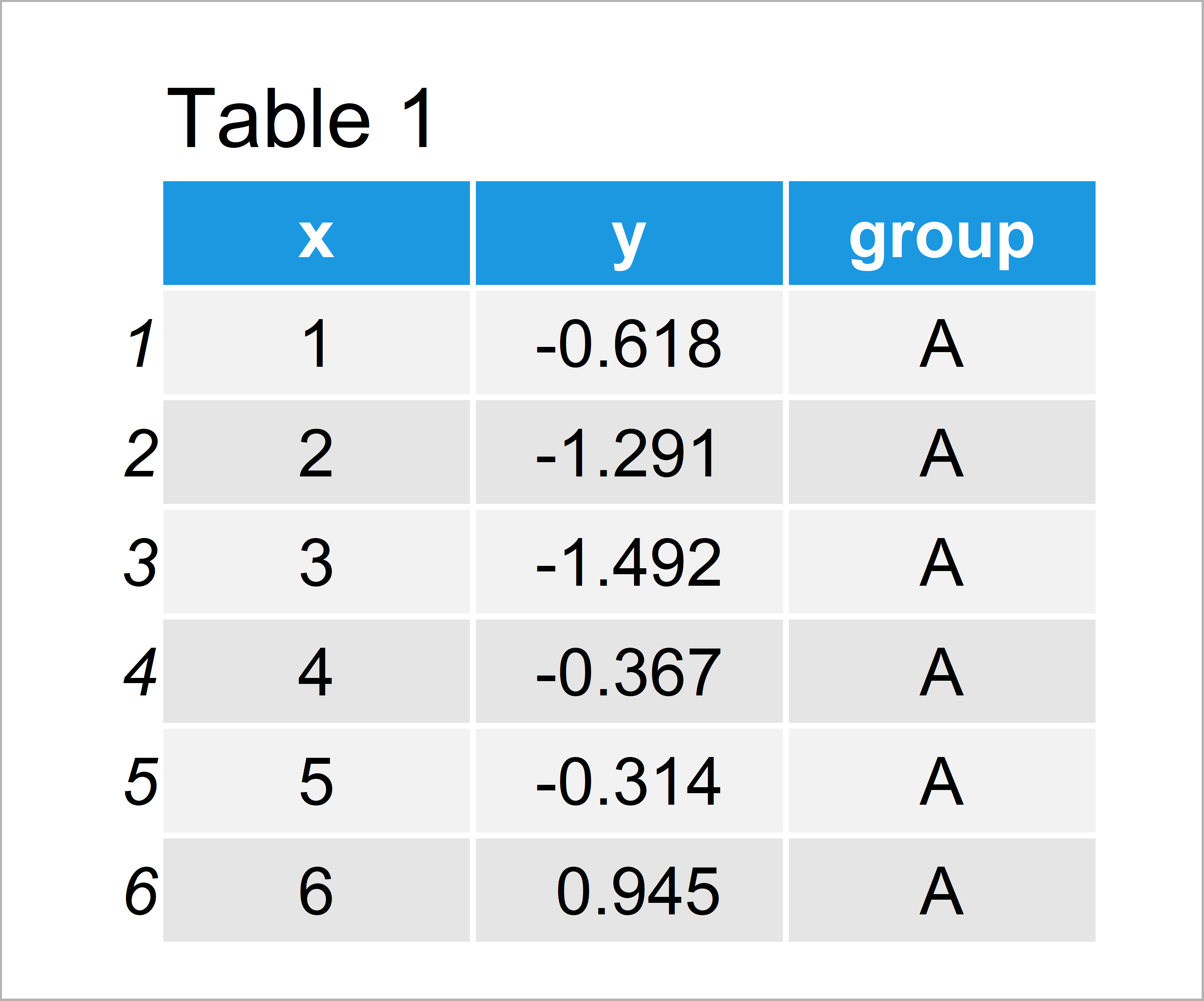# Add Labels at Ends of Lines in ggplot2 Line Plot in R (Example)

In this tutorial you’ll learn how to draw a ggplot2 line graph with labels at the end of each line in the R programming language.

The tutorial contains these content blocks:

So let’s get started!

## Example Data, Add-On Packages & Basic Plot

Consider the exemplifying data below:

```set.seed(2923874)                               # Create example data
data <- data.frame(x = 1:10,
y = c(rnorm(10),
rnorm(10, 3, 3),
rnorm(10, 10, 1.5),
rnorm(10, 6, 2)),
group = rep(LETTERS[1:4], each = 10))
head(data)                                      # First six rows of example data```Table 1 shows the structure of our example data: It contains 40 rows and three columns.

We also need to install and load the ggplot2 package, if we want to use the corresponding functions:

```install.packages("ggplot2")                     # Install & load ggplot2
library("ggplot2")```

As next step, we can create a line graphic of our data using the ggplot2 package:

```ggplot(data, aes(x, y, col = group)) +          # Draw default ggplot2 plot
geom_line()```In Figure 1 you can see that we have created a ggplot2 line chart with four different lines. On the right side of the plot, you can see the default legend of the ggplot2 package.

## Example: Draw Labels at Ends of Lines in ggplot2 Line Plot Using ggrepel Package

The following R programming code shows how to add labels at the ends of the lines in a ggplot2 line graph.

As a first step, we have to add a new column to our data that contains the text label for each line in the row with the maximum value on the x-axis:

```data_label <- data                              # Modify data
data_label\$label <- NA
data_label\$label[which(data_label\$x == max(data_label\$x))] <- data_label\$group[which(data_label\$x == max(data_label\$x))]```

Furthermore, we have to install and load the ggrepel package to RStudio:

```install.packages("ggrepel")                     # Install ggrepel package

Next, we can use the geom_label_repel function of the ggrepel package to add labels at the end of each line. Note that we are also removing the default ggplot2 legend by specifying theme(legend.position = “none”).

```ggplot(data_label, aes(x, y, col = group)) +    # Draw ggplot2 plot with labels
geom_line() +
geom_label_repel(aes(label = label),
nudge_x = 1,
na.rm = TRUE) +
theme(legend.position = "none")```Figure 2 shows the output of the previous code: A ggplot2 line plot with labels at the ends of lines.

## Video, Further Resources & Summary

Would you like to know more about line plots in R? Then you may want to have a look at the following video of my YouTube channel. I illustrate the R code of this article in the video tutorial.

Please accept YouTube cookies to play this video. By accepting you will be accessing content from YouTube, a service provided by an external third party.If you accept this notice, your choice will be saved and the page will refresh.

Furthermore, you might want to have a look at the related posts of this website. I have published several articles about graphics in R already.

On this page you have learned how to add text labels to each line of a ggplot2 line chart in the R programming language. If you have additional questions, tell me about it in the comments section.

Subscribe to the Statistics Globe Newsletter

•Manal
September 27, 2022 3:06 am

thank you for the explanation it’ really helpful.
I follow your code, but I end up with having an error when I run the geom_label_repel()
Error in grid.Call(C_convert, x, as.integer(whatfrom), as.integer(whatto), :
Viewport has zero dimension(s)
Removed 42 rows containing missing values (geom_label_repel).

Any idea how to solve that

•September 30, 2022 5:20 pm